IdentifiantMot de passe
Mot de passe oublié ?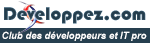Vous êtes nouveau sur Developpez.com ? Créez votre compte ou connectez-vous afin de pouvoir participer !

Vous devez avoir un compte Developpez.com et être connecté pour pouvoir participer aux discussions.

Vous n'avez pas encore de compte Developpez.com ? Créez-en un en quelques instants, c'est entièrement gratuit !

Si vous disposez déjà d'un compte et qu'il est bien activé, connectez-vous à l'aide du formulaire ci-dessous.

##### Identifiez-vous
 Identifiant Mot de passe Mot de passe oublié ?
##### Créer un compte

L'inscription est gratuite et ne vous prendra que quelques instants !

# Le Top 32 des algorithmes les plus importants au monde Lesquels comprenez-vous et utilisez-vous ?

Le , par Katleen Erna

0PARTAGES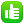2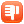1
Le Top 32 des algorithmes les plus importants au monde, lesquels comprenez-vous et utilisez-vous ?

Un blogueur américain a posté un billet dans lequel il explique avoir essayé avec ses collègues de répertorier les algorithmes les plus importants au monde. Après un gros brainstorming, ces passionnés ont établi une liste de 32 entrées.

Leur critère ? Qu'il s'agisse d'algorithmes très largement utilisés en informatique et en mathématique.

Voici leur liste :

A* search algorithm
Graph search algorithm that finds a path from a given initial node to a given goal node. It employs a heuristic estimate that ranks each node by an estimate of the best route that goes through that node. It visits the nodes in order of this heuristic estimate. The A* algorithm is therefore an example of best-first search.

Beam Search
Beam search is a search algorithm that is an optimization of best-first search. Like best-first search, it uses a heuristic function to evaluate the promise of each node it examines. Beam search, however, only unfolds the first m most promising nodes at each depth, where m is a fixed number, the beam width.

Binary search
Technique for finding a particular value in a linear array, by ruling out half of the data at each step.

Branch and bound
A general algorithmic method for finding optimal solutions of various optimization problems, especially in discrete and combinatorial optimization.

Buchberger's algorithm
In computational algebraic geometry and computational commutative algebra, Buchberger's algorithm is a method of transforming a given set of generators for a polynomial ideal into a Gröbner basis with respect to some monomial order. One can view it as a generalization of the Euclidean algorithm for univariate gcd computation and of Gaussian elimination for linear systems.

Data compression
Data compression or source coding is the process of encoding information using fewer bits (or other information-bearing units) than an unencoded representation would use through use of specific encoding schemes.

Diffie-Hellman key exchange
Cryptographic protocol which allows two parties that have no prior knowledge of each other to jointly establish a shared secret key over an insecure communications channel. This key can then be used to encrypt subsequent communications using a symmetric key cipher.

Dijkstra's algorithm
Algorithm that solves the single-source shortest path problem for a directed graph with nonnegative edge weights.

Discrete differentiation
I.e., the formula f'(x) = (f(x+h) - f(x-h)) / 2h.

Dynamic programming
Dynamic programming is a method for reducing the runtime of algorithms exhibiting the properties of overlapping subproblems and optimal substructure, described below.

Euclidean algorithm
Algorithm to determine the greatest common divisor (gcd) of two integers. It is one of the oldest algorithms known, since it appeared in Euclid's Elements around 300 BC. The algorithm does not require factoring the two integers.

Expectation-maximization algorithm (EM-Training)
In statistical computing, an expectation-maximization (EM) algorithm is an algorithm for finding maximum likelihood estimates of parameters in probabilistic models, where the model depends on unobserved latent variables. EM alternates between performing an expectation step, which computes the expected value of the latent variables, and a maximization step, which computes the maximum likelihood estimates of the parameters given the data and setting the latent variables to their expectation.

Fast Fourier transform (FFT)
Efficient algorithm to compute the discrete Fourier transform (DFT) and its inverse. FFTs are of great importance to a wide variety of applications, from digital signal processing to solving partial differential equations to algorithms for quickly multiplying large integers.

Gradient descent is an optimization algorithm that approaches a local minimum of a function by taking steps proportional to the negative of the gradient (or the approximate gradient) of the function at the current point. If instead one takes steps proportional to the gradient, one approaches a local maximum of that function; the procedure is then known as gradient ascent.

Hashing
A function for summarizing or probabilistically identifying data. Typically this means one applies a mathematical formula to the data, producing a string which is probably more or less unique to that data. The string is much shorter than the original data, but can be used to uniquely identify it.

Heaps (heap sort)
In computer science a heap is a specialized tree-based data structure. Heaps are favourite data structures for many applications: Heap sort, selection algorithms (finding the min, max or both of them, median or even any kth element in sublinear time), graph algorithms.

Karatsuba multiplication
For systems that need to multiply numbers in the range of several thousand digits, such as computer algebra systems and bignum libraries, long multiplication is too slow. These systems employ Karatsuba multiplication, which was discovered in 1962.

LLL algorithm
The Lenstra-Lenstra-Lovasz lattice reduction (LLL) algorithm is an algorithm which, given a lattice basis as input, outputs a basis with short, nearly orthogonal vectors. The LLL algorithm has found numerous applications in cryptanalysis of public-key encryption schemes: knapsack cryptosystems, RSA with particular settings, and so forth.

Maximum flow
The maximum flow problem is finding a legal flow through a flow network that is maximal. Sometimes it is defined as finding the value of such a flow. The maximum flow problem can be seen as special case of more complex network flow problems. The maximal flow is related to the cuts in a network by the Max-flow min-cut theorem. The Ford-Fulkerson algorithm computes the maximum flow in a flow network.

Merge sort
A sorting algorithm for rearranging lists (or any other data structure that can only be accessed sequentially, e.g. file streams) into a specified order.

Newton's method
Efficient algorithm for finding approximations to the zeros (or roots) of a real-valued function. Newton's method is also a well-known algorithm for finding roots of equations in one or more dimensions. It can also be used to find local maxima and local minima of functions.

Q-learning
Q-learning is a reinforcement learning technique that works by learning an action-value function that gives the expected utility of taking a given action in a given state and following a fixed policy thereafter. A strength with Q-learning is that it is able to compare the expected utility of the available actions without requiring a model of the environment.

The quadratic sieve algorithm (QS) is a modern integer factorization algorithm and, in practice, the second fastest method known (after the number field sieve, NFS). It is still the fastest for integers under 110 decimal digits or so, and is considerably simpler than the number field sieve.

RANSAC
RANSAC is an abbreviation for "RANdom SAmple Consensus". It is an algorithm to estimate parameters of a mathematical model from a set of observed data which contains "outliers". A basic assumption is that the data consists of "inliers", i. e., data points which can be explained by some set of model parameters, and "outliers" which are data points that do not fit the model.

RSA
Algorithm for public-key encryption. It was the first algorithm known to be suitable for signing as well as encryption. RSA is still widely used in electronic commerce protocols, and is believed to be secure given sufficiently long keys.

Schönhage-Strassen algorithm
In mathematics, the Schönhage-Strassen algorithm is an asymptotically fast method for multiplication of large integer numbers. The run-time is O(N log(N) log(log(N))). The algorithm uses Fast Fourier Transforms in rings.

Simplex algorithm
In mathematical optimization theory, the simplex algorithm a popular technique for numerical solution of the linear programming problem. A linear programming problem consists of a collection of linear inequalities on a number of real variables and a fixed linear functional which is to be maximized (or minimized).

Singular value decomposition (SVD)
In linear algebra, SVD is an important factorization of a rectangular real or complex matrix, with several applications in signal processing and statistics, e.g., computing the pseudoinverse of a matrix (to solve the least squares problem), solving overdetermined linear systems, matrix approximation, numerical weather prediction.

Solving a system of linear equations
Systems of linear equations belong to the oldest problems in mathematics and they have many applications, such as in digital signal processing, estimation, forecasting and generally in linear programming and in the approximation of non-linear problems in numerical analysis. An efficient way to solve systems of linear equations is given by the Gauss-Jordan elimination or by the Cholesky decomposition.

Strukturtensor
In pattern recognition: Computes a measure for every pixel which tells you if this pixel is located in a homogenous region, if it belongs to an edge, or if it is a vertex.

Union-find
Given a set of elements, it is often useful to partition them into a number of separate, nonoverlapping groups. A disjoint-set data structure is a data structure that keeps track of such a partitioning. A union-find algorithm is an algorithm that performs two useful operations on such a data structure:
Find: Determine which group a particular element is in.
Union: Combine or merge two groups into a single group.

Viterbi algorithm
Dynamic programming algorithm for finding the most likely sequence of hidden states - known as the Viterbi path - that result in a sequence of observed events, especially in the context of hidden Markov models.
Source : http://www.risc.jku.at/people/ckouts...lgorithms.html The Most Important Algorithms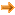Parmi ces algorithmes, lesquels connaissez-vous et lesquels utilisez-vous ?Comprenez-vous tous ces algorithmes ?Si vous aviez participé au brainstorming original, auriez-vous ajouté une autre entrée à cette liste ? Pourquoi ?

Une erreur dans cette actualité ? Signalez-nous-la !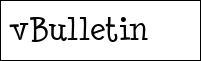Membre actif
Le 06/07/2010 à 9:58
Bonjour,
cette liste me parait assez bizarre de par le statut des éléments qui la composent. D'une part on a de vrais algorithmes (Dijkstra, dérivation discrète, Karatsuba) et d'autres part on a des méthodes tellement générales que cela a peu de sens (programmation dynamique, compression).
On peut remarquer par ailleurs que la dernière entrée (Viterbi) rentre dans la catégories de la programmation dynamique.
Bon j'arrête là de faire mon rabat-joie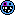Je connais bien la programmation dynamique, Karatsuba, Dijkstra, Ford-Fulkerson, le tri fusion et d'autres. Je connais la plupart au moins de noms mais ça s'arrête là pour certains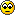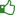3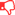0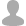Membre expérimenté
Le 06/07/2010 à 9:27
Dommage que ça ne soit pas en français, ça a l'air intéressant...42Rédacteur/Modérateur
Le 07/07/2010 à 3:45
Y a pas le Quicksort dans leur liste ??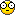C'est quand même un des algos les plus connus et les plus utilisés au monde, certainement beaucoup plus que certains qui sont mentionnés et que quasiment personne ne connait... Sérieusement, combien de personnes ont déjà entendu parler de "Karatsuba multiplication", et combien connaissent le Quicksort ? A mon avis y a pas photo...20Membre expérimenté
Le 07/07/2010 à 19:37
Il est vrai qu'on y trouve de tout et n'importe quoi et surtout des trucs qui ne sont pas ou peu utilisés, même dans les domaines d'où ils découlent.
Ensuite c'est clair qu'ils ont visiblement confondu Algorithmes et Methodologie ou Modélisation, car effectivement Dynamic Programming n'a rien à faire dans cette liste vraiment très light.

Mais pire, il n'y a pas de classement dans le sens du plus utilisé au moins utilisé, Djisktra est probablement le plus utilisé sans que personne ne le sache vraiment, car il est à la base d'OSPF sans quoi vous pourriez dire adieu à internet, car plus de routage...
D'ailleurs, il reste la référence en réseau, nettement plus que A* même s'il est aussi très utilisé, puisqu'internet ce n'est jamais qu'un vaste GrapheDiffie-Hellman est utilisé certes, mais pas tant que cela au regard des grands absents que sont Quick Sort ou d'autres algorithmes de tri rapides comme Heap Sort.

En réalité, leurs choix ne sont fondés sur rien si ce n'est peut-être le nombre d'occurrences sur le net et notamment des algorithmes pas utilisés car mauvais mais qui justement ont fait énormément couler d'encre...

En plus leur liste ne peut refléter que leur sensibilité à eux, dans la mesure où la sensibilité Européenne est toute autre, et certains algorithmes notamment en développement sont fortement utilisés aux usa et pas ici, et inversement, pour des raisons diverses et variées toutes meilleures les unes que les autres.

Il aurait été plus critique et cohérent de faire une liste par domaine d'application quitte à réduire le nombre d'algorithme par domaine, comme un top 10 de chaque, cette approche aurait été nettement plus crédible, et moins fourre tout, car là comme je le disais, on se demande vraiment sur quels faits, ou mêmes quelles statistiques tangibles ils se fondent pour avancer leurs choix.20Membre averti
Le 08/07/2010 à 9:42Envoyé par danbo52Bon, j'ai pas que ça à faire, faut que j'aille faire une réduction de matrice pour un problème d'optimisation volumétrique de chargement de container avec des objets de formes bizarres (en gros,, j'ai des objets tordus, combien et comment je les mets dans mon container, pour optimiser les coûts...)

Bonne cérébralisation à tous.
En fait tu joues à Tetris 3D ?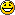20Membre à l'essai
Le 06/07/2010 à 7:19
Hé ben ! Il m'en reste pas mal à découvrirJe connais et maîtrise l'A*,Dijkstra, la méthode du Simplex , le tri - fusion et que j'exploite essentiellement en cours et parfois dans des projets personnelles, ainsi que l'algorithme Union - Find et le calcul de Flot maximum que j'avais vu dans un bouquin ^^ (sans compter celui d'Euclide que j'ai appris au lycée).

Après je connais certains grand nom comme la transformé de fourier utilisé en réseaux il me semble (les sales souvenirs de matlab à utiliser ça en cours sans savoir ce que c'est) et le RSA. Mais quand même il y en a un paquet que je connais pas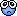En revanche la programmation dynamique n'est pas un algorithme je vois pas ce que ça fait dans cette liste, c'est une branche d'algorithme pas un algorithme proprement dit =/10Membre confirmé
Le 06/07/2010 à 8:44
Ca rappelle pas mal de trucs vu en cours cette liste. Je pensais pas un jour tomber à nouveau sur ces termes que j'avais considéré comme "à oublier le lendemain de l'examen" (remarque... comme la plupart des choses que j'ai apprises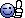).

Y a quand même quelques trucs marrants, genre :

In computational algebraic geometry and computational commutative algebra, Buchberger's algorithm is a method of transforming a given set of generators for a polynomial ideal into a Gröbner basis with respect to some monomial order. One can view it as a generalization of the Euclidean algorithm for univariate gcd computation and of Gaussian elimination for linear systems
Un seul mot à répondre à ça : WTF ?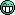10Modérateur
Le 07/07/2010 à 12:43
C'est absolument pas crédible, ni exhaustif !!!
Si on en croit leurs critères, il manque deux algorithmes incontournable d'imagerie :
- Bresenham => utilisé dans TOUTES les cartes graphiques. Donc plus utilisé que ça tu meurs.
- Z-Buffer => utilisé dans TOUTES les cartes graphiques.10Candidat au Club
Le 07/07/2010 à 13:00
tres jolie,
il me reste pas mal d'algorithme a voir.10Membre éprouvé
Le 07/07/2010 à 13:48
Salut,

On retrouve les tartes à la crème du calcul numérique (FFT, Euclide, dérivée discrète, Newton ...).

Les poids lourds de la crypto et du codage (RSA, Hash-coding, ...)

Je rejoins l'avis de grand nombre d'entre-vous : certains "algo" présentés ne sont pas des algo mais plutôt des domaines d'application (data compression, programmation dynamique, ...)

On aurait pu ajouter par exemple l'algorithme de code correcteur d'erreur de Reed-Salomon qui est particulièrement utilisé dans la lecture des CD Audio (le fait de lire sans erreur un CD rayé au tampon Jex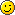)

Dans dans le domaine plus calculatoire : les algo différences finie : euler, RK2/4, ...

Et bien sûr Quicksort, le grand absent.

Mais bon, difficile de juger de l'intérêt d'une telle liste au vu de la grande variété des domaines d'applications....

En tous cas, de sacrés souvenirs sur les bancs des facsB.10